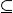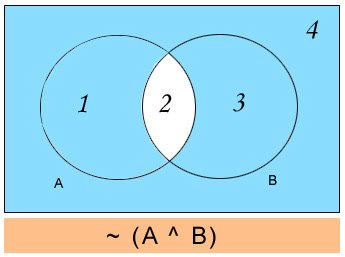# Set Theory

Basics of Set Theory

Set Theory is considered as a
part of modern mathematics that helps in developing a fruitful research area of
its own. Set theory had its origin in work done by Georg Cantor in the late 19th
century mainly on certain kinds of infinite series called Fourier series. From
this point of view, most mathematicians accept set theory as a foundation for
mathematics. The set notions and the membership in a set can be used as the
most primitive ideas in terms of which all-mathematical objects and ideas can
be defined.

Basic Concepts (Signs/
Notation/ Explanation)

Set Theory begins with a
fundamental binary relation between an object and set. For instance if A is a
set and x is an element of A, we write x â‚¬ A. A binary relation between two set
can also be a subset relation also called Set inclusion. This happens when all
the members of set A are also members of set B, then A is the subset of B, well
denoted by AÂ B. For instance, {1,2} is
a subset of {1,2,3} but {1,4} is not. Hence, right from this definition it is
clear that a set is the subset of itself.

Two sets are said to be equal if
and only if they have the same elements. Thus for example {1, 2, 3} = {3, 2, 1}
that means the order of elements does not matter.

Similarly, in understanding subsets,
set A is a subset of a set B when everything in A is also in B. More
properly, for sets A and B, A is a proper subset of B and denoted by AÂ B, when
Â AÂ B
and A â‰  B, For example {1, 2}{3, 2, 1}

In cardinality, if a set S has n
distinct element for some natural number n, then n is the cardinality of S and
S is a finite set. For example, the cardinality of the set {3, 1, 2} is 3.

Empty or null set needs no
mentioning. More formally, an empty set is denoted by Ã˜.Â  Note that Ã˜ and {Ã˜}
are different sets. {Ã˜} has one element namely Ã˜ in it. So, {Ã˜} is not empty.
But, Ã˜ has nothing in it.

Universal set is a set, which has
all the elements in the universe of discourse. More properly, a universal set
denoted by, denoted by U.

Rules

Letâ€™s say that our universe contains
the numbers 1, 2, 3, and 4. Let A be the set containing the numbers 1 and 2,
that is A= {1, 2} and let B be the set containing the numbers 2 and 3; that is B
= {2, 3}. Here are the following relationships with blue shade, marking the
solution region in the Venn diagrams.

If the set notation is A U B then
it would be everything that falls in either of the sets. Here the answer will
be {1, 2, 3}If the set notation is A
intersection B or Aâˆ©B, it means the notation is referring to the elements
that are common in both of the sets. Here the answer will be {2}

ÂIf the set notation is ~ A. This
means all elements in the universe outside of A. Here the answer will be {3,
4}.

ÂIf the set notation is A- B, (i.e
A minus B or A compliment B), it means everything in A except for anything in
its overlap with B. Here the answer will be {1}.If the set notation is ~ (A U B),
i.e not (A union B). This denotes everything that falls outside A and B. HereIf the set notation is ~ (A ^ B)
it means everything outside the overlapping region of A and B giving answer
like {1, 3, 4}.

ÂAs you can see above a subset is
a set, which is totally contained within another set. For instance, every set
in a Venn Diagram is a subset of the diagramâ€™s universe.Venn diagram can also demonstrate
disjoint sets. In the above graphic representation, you will find A and B are
disjoint. As disjoint sets have no overlapping, so this means their
intersection is empty.

However, we can follow some other
ways to describe sets. When the set is a small finite set or an infinite set
whose elements can be referred to using “â€¦” For example

A= {1, 2}

N= {1, 2, 3â€¦} These are all set
of natural numbers. Again, there are some people who include “0” in what they
call the set of natural numbers. W= {0, 1, 2â€¦} that are known as the set of
whole numbers while Z = {0, Â±1, Â± 2…}. These are known as set of integers.

Concepts like Union,
Intersections and compliments

Just as arithmetic features
binary operations on numbers, set theory features binary operations on sets.

– Union of set A and B denoted AU
B is the set whose members are members of at least one of A or B. The union of
{1, 2, 3} and {2, 3, 4} is the set {1, 2, 3, 4}

– Intersection of sets A and B, denoted
by A âˆ© B is the set whose members are both A and B. The intersection of
{1, 2, 3} and {2, 3, 4} is the set {2, 3}

– Compliment of set A relative to Set U denoted by A c
is the set of all members of U that are not members of A. This term is commonly
employed when U is a universal set. This operation is also called the set
difference of U and A, denoted by U \ A. For example the compliment of {1,2,3}
relative to {2,3,4}is {4} while the compliment of {2,3,4} relative to {1,2,3}
is {1}.